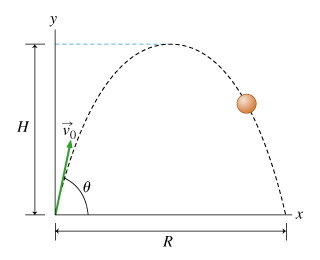# Problem: c) Find H, the maximum height attained by the projectile. Express the time in terms of v0, θ, and g. d) Find the total distance R (often called the range) traveled in the x-direction; see the figure in the problem introduction.

###### FREE Expert Solution

c)

Using the kinematics equation:

$\overline{){{{\mathbf{v}}}_{{\mathbf{f}}{\mathbf{y}}}}^{{\mathbf{2}}}{\mathbf{=}}{{{\mathbf{v}}}_{{\mathbf{0}}{\mathbf{y}}}}^{{\mathbf{2}}}{\mathbf{+}}{\mathbf{2}}{\mathbf{a}}{\mathbf{\Delta }}{\mathbf{y}}}$

96% (458 ratings)###### Problem Detailsc) Find H, the maximum height attained by the projectile. Express the time in terms of v0, θ, and g.

d) Find the total distance R (often called the range) traveled in the x-direction; see the figure in the problem introduction.